# SQL Server 2019 Extensibility Framework & Java - Null Values

This post is the third post in a series where I look at the Java extension in SQL Server, i.e. the ability to execute Java code from inside SQL Server.

To see what other posts there are in the series, go to SQL Server 2019 Extensibility Framework & Java.

In this post we look at something related to the data passing post; how to handle null values.

DISCLAIMER: This post contains Java code. I am not a Java guy, in fact, the only Java I have ever written is the code in this post and the previous SQL Server 2019 Java posts. So, the code is not elegant in any shape or form, and I am absolutely certain it can be done in a much better way. However, this is not about Java as such, but how you call Java code from SQL Server, and what you need to implement on the Java side.

Before we dive into this post’s topic, let us do a recap.

## Recap

In the SQL Server 2019 Extensibility Framework & Java - Passing Data post, we looked at how we pass data back and forth between SQL Server and Java. In the Java extensions we do not have the `InputDataSet`, and `OutputDataSet` variables, so we need to define class member arrays for the columns we send in and pass back out, as well as a variable indicating the number of columns we return:

• `inputDataCol``N`: array variable representing the input columns, where N is the column number (1 based).
• `outputDataCol``N`: array variable representing the output columns, where N is the column number (1 based).
• `numberofOutputCols`: it represents the number of columns returned from Java, and it is always required - regardless if you return columns or not.

In addition to these variables we need two variables mapping null values:

• `inputNullMap`: two dimensional `boolean` array variable, indicating whether a column value is `null`.
• `outputNullMap`: two dimensional `boolean` array variable, indicating whether a column value is `null`.

All the `input``xxx` variables get populated automatically, whereas you need to populate the `output``xxx` variables in the code.

In the post we had some example code looking like so:

``````public class DataPassing {
//input data variables
static public int[] inputDataCol1 = new int;
static public int[] inputDataCol2 = new int;
static public int[] inputDataCol3 = new int;
static public boolean[][] inputNullMap = new boolean;

//output variables
static public int[] outputDataCol1;
static public int[] outputDataCol2;
static public int[] outputDataCol3;
static public boolean[][] outputNullMap;

static public short numberOfOutputCols;

public static void bar() {

int numRows = inputDataCol1.length;
numberOfOutputCols = 3;

outputDataCol1 = new int[numRows];
outputDataCol2 = new int[numRows];
outputDataCol3 = new int[numRows];

for(int x = 0; x < numRows; x++) {
outputDataCol1[x] = inputDataCol1[x];
outputDataCol2[x] = inputDataCol2[x];
outputDataCol3[x] = inputDataCol3[x];
}

outputNullMap = new boolean[numberOfOutputCols][numRows];
}

}
``````

Code Snippet 1: Example Code

The code we see in Code Snippet 1 represents a class to which we pass in a data set consisting of three columns. The class passes back a three column data set. What the code does should be pretty self-explanatory, but there are two array variables that we are not doing much with: `inputNullMap` and `outputNullMap`, and today we look at them.

## Demo Data

In today’s post, we use some data from the database, so let us set up the necessary database, a table, and load data into the table:

``````USE master;
GO
SET NOCOUNT ON;
GO
DROP DATABASE IF EXISTS JavaNullDB;
GO
CREATE DATABASE JavaNullDB;
GO
USE JavaNullDB;
GO
DROP TABLE IF EXISTS dbo.tb_NullRand10
CREATE TABLE dbo.tb_NullRand10(RowID int identity primary key,
x int, y int, col1 nvarchar(50));
GO
INSERT INTO dbo.tb_NullRand10(x, y, col1)
SELECT TOP(10) CAST(ABS(CHECKSUM(NEWID())) % 14 AS int)
, CAST(ABS(CHECKSUM(NEWID())) % 20 AS int)
, N'Hello ' + CAST(CAST(ABS(CHECKSUM(NEWID())) % 25 AS int) AS nvarchar(50))
FROM sys.objects o1
CROSS JOIN sys.objects o2
CROSS JOIN sys.objects o3
CROSS JOIN sys.objects o4;
GO
UPDATE dbo.tb_NullRand10
SET y = NULL
WHERE RowId = 3
UPDATE dbo.tb_NullRand10
SET Col1 = NULL
WHERE RowId = 5
UPDATE dbo.tb_NullRand10
SET x = NULL
WHERE RowId = 6
UPDATE dbo.tb_NullRand10
SET y = NULL
WHERE RowId = 8
UPDATE dbo.tb_NullRand10
SET col1 = NULL
WHERE RowId = 9
GO
``````

Code Snippet 2: Create Database Objects

We see from Code Snippet 2 how we:

• Create a database: `JavaNullDB`.
• Create a table: `dbo.tb_NullRand10`.
• Insert some data into the table.

The data we insert is entirely random, but it gives us something to “play around” with. Now, when we have a database and some data let us get started.

## Null Values

So why do we care about null values? Well, the reason is that in SQL Server all data types are nullable, whereas in Java that is not the case. In Java, like in .NET, primitive types (`int`, etc.) cannot be null, so code like this:

``````public static void foo() {
int x;
x = null;
}
``````

Code Snippet 3: Null Value

When we try and compile the code in Code Snippet 3 we get a compile-time error: `error: incompatible types: <null> cannot be converted to int`. In this case, the compiler saves us, but if we now think about passing in data from SQL Server, we can get into trouble as columns in the dataset can be null. So what do we do?

In the SQL Server 2019 Java Extension there are a couple of things helping with null values:

• Extension components.
• Null maps.

#### Extension Components

In previous posts, I have briefly mentioned the Java extension components. They are similar to the launchers, and the “link” dll’s for R/Python, and they are involved when passing data to Java as well as receiving data from Java.

NOTE: I cover these components in future posts.

Let us try to get an understanding of what the components do when passing data to Java. We start with doing a simple `SELECT RowID, x, y FROM dbo.tb_NullRand10`, where `x` and `y` are integer columns: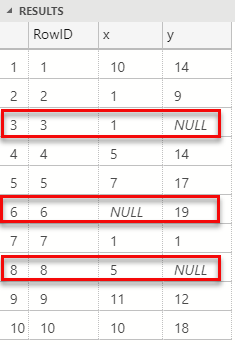Figure 1: Select with NULL

In Figure 1, (highlighted in red), we see, which we also mentioned above, how primitive types are nullable in SQL. However, let us say we have some Java code looking like so:

``````public class NullValues {
static public int[] inputDataCol1 = new int;
static public int[] inputDataCol2 = new int;
static public int[] inputDataCol3 = new int;
static public boolean[][] inputNullMap = new boolean;

static public short numberOfOutputCols;

public static void foo() {

for(int x = 0; x < inputDataCol1.length; x++) {
System.out.printf("%d\t\t%d\t\t%d\n",
inputDataCol1[x],
inputDataCol2[x],
inputDataCol3[x]);
}
}
}
``````

Code Snippet 4: Java Code

In the code in Code Snippet 4 we see how we expect a three-column dataset passed in, which we then print out in the `foo` method. The immediate problem is that, as we see in Figure 1, the dataset consists of null values, so what happens if we execute some SQL code looking like so:

``````EXEC sp_execute_external_script
@language = N'Java'
, @script = N'NullValues.foo'
, @input_data_1 = N'SELECT RowID, x, y FROM dbo.tb_NullRand10';
``````

Code Snippet 5: SQL Pushing in Null Values

When we run the code in Code Snippet 5 the result looks as follows: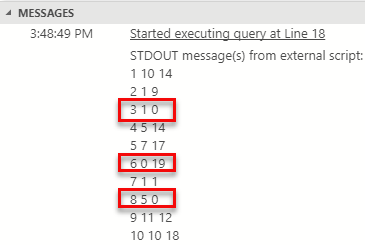Figure 2: Dataset with Null Values in Java

Ok, we did not crash and burn, that is good! However, what about the rows with the null values, `RowId`’s 3, 6, and 8? Notice in Figure 2 how the values printed out are set to 0 where we in Figure 1 saw nulls. So something has “automagically” converted the nulls to 0’s. That something is one of the Java extension components, and from a high level it works something like so (this is somewhat of guesswork from me):

1. SQL Server pushes the data into the component.
2. The component loops through the data and replaces null values with the data type’s default value.
3. The component calls into the relevant class and method and copies the data into the column variables.

That is nice, but now we receive 0’s instead for nulls, what about if we want to handle null differently than 0. Think about how SQL Server handles null in some of its functions; columns with null values are ignored. With nulls replaced with 0’s, what do we do?

#### Null Maps

Null maps solve the problem of null values coming back with the default value for the type. Remember what we said in the previous post and in the Recap above that null maps are two-dimensional arrays indicating whether a value is null or not, and that we have two null maps:

• `inputNullMap`: for data passed in.
• `outputNullMap`: for data returned.

inputNullMap

For input data we use the `inputNullMap`, or rather one of the Java extension components populates the map, and we use it in our code like so:

``````public static void bar() {

int numRows = inputDataCol1.length;
int numCols = inputNullMap.length;
for(int x = 0; x < numRows; x++) {
for(int y = 0; y < numCols; y++) {
System.out.printf("Null map value at row: %d, column: %d, value: %b\n",
x, y, inputNullMap[y][x]);
}
}
}
``````

Code Snippet 6: Input Data Null Map

We see in Code Snippet 6 how we have a new method: `bar`, where we loop through the `inputNullMap` array. For each row, we loop the columns and print out the boolean value indicating if a column value is null or not.

Let us change the code in Code Snippet 5 to call into the `bar` method:

``````EXEC sp_execute_external_script
@language = N'Java'
, @script = N'NullValues.bar'
, @input_data_1 = N'SELECT RowID, x, y
FROM dbo.tb_NullRand10
WHERE RowID IN(3, 6, 8)';
``````

Code Snippet 7: SQL Pushing in Null Values - II

Apart from calling into the `bar` method in Code Snippet 7 the other difference from Code Snippet 5 is that we only push in rows which have null values, (to keep it short). The result we see after we execute looks like this:Figure 3: Null Map Output

We see, highlighted in yellow in Figure 3, how the null map indicates which columns have null values.

So how do we use this? Let us assume we have code which takes a dataset and multiplies two columns together (`x` and `y`) and returns the `RowID` and the result back to the caller:

``````public class NullValues {
static public int[] inputDataCol1 = new int;
static public int[] inputDataCol2 = new int;
static public int[] inputDataCol3 = new int;
static public boolean[][] inputNullMap = new boolean;

static public int[] outputDataCol1;
static public int[] outputDataCol2;
static public boolean[][] outputNullMap;

static public short numberOfOutputCols;

public static void multiplier() {

int numRows = inputDataCol1.length;

outputDataCol1 = new int[numRows];
outputDataCol2 = new int[numRows];

for(int i = 0; i < numRows; i++) {
outputDataCol1[i] = inputDataCol1[i];
outputDataCol2[i] = inputDataCol2[i] * inputDataCol3[i];

}

numberOfOutputCols = 2;
outputNullMap = new boolean[numberOfOutputCols][numRows];

}
...
}
``````

Code Snippet 8: Multiplier

We see in Code Snippet 8 how we:

• Declare two output column arrays for the two column output dataset.
• Declare an output null map.
• In the `multiplier` method initialize the output column arrays.

We then loop through the input data and assigns the `RowID` (`inputDataCol1`) to `outputDataCol1`, and sets the value of `outputDataCol2` to be `inputDataCol2 * inputDataCol3`. If we at this stage compiled, moved the `.class` file to the `CLASSPATH` location, and executed as per Code Snippet 5 (obviously edit the `@script` variable to be: `@script = N'NullValues.multiplier'`), this is the result: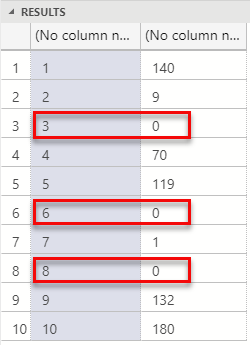Figure 4: Multiplier Output

What we see in Figure 4 is how the rows with original null values came back with a result of 0 as, as we know from above, the Java components replace null with 0. However, this may not be what we want; instead we want the original null value columns not to be part of the result at all. So, to not include the original null value columns we use the input null map:

``````import java.util.ArrayList;
import java.util.stream.*;
import java.util.*;

public class NullValues {
//static variables as in Code Snippet 8 ...

public static void multiplier2() {

int numRows = inputDataCol1.length;
int numCols = inputNullMap.length;

List<Integer> rowId = new ArrayList<Integer>();
List<Integer> result = new ArrayList<Integer>();

Boolean colIsNull;

for(int i = 0; i < numRows; i++) {
colIsNull = false;
for(int y = 0; y < numCols; y++) {
if(inputNullMap[y][i] == true) {
colIsNull = true;
break;
}
}
if(!colIsNull) {
}
}

int numOutRows = rowId.size();
outputDataCol1 = new int[numOutRows];
outputDataCol2 = new int[numOutRows];

for(int i = 0; i < numOutRows; i++) {
outputDataCol1[i] = rowId.get(i);
outputDataCol2[i] = result.get(i);
}
numberOfOutputCols = 2;
outputNullMap = new boolean[numberOfOutputCols][numOutRows];
}

// the other methods ...
}
``````

Code Snippet 9: Using Input Null Map for Output Data

So in Code Snippet 9 we now see a couple of different things compared with Code Snippet 8:

• As we do not know upfront how many rows the method returns, we use Java `List`’s to load output data into. The two lists are for the `RowID` and the result output columns.
• We use the `inputNullMap` to check if any column in the row we process is null. If so we break immediately out, and we go to next row.
• If the columns for the row is not null, we add the `RowID` column (`inputDataCol1`) to the row id list: `rowId`.
• If none of the columns in the row are null we do the multiplication of `x` and `y`, (`inputDataCol2`, `inputDataCol3`), and add the result to the `result` list.
• We initialize the two output data column arrays with the size of the `rowId` list.
• Finally we loop over the data in the two lists and add it to the two output data column arrays.

When we now execute the code in Code Snippet 5, (after compiling etc.), and having changed the @script parameter to: `@script = N'NullValues.multiplier2'`, the result is as so: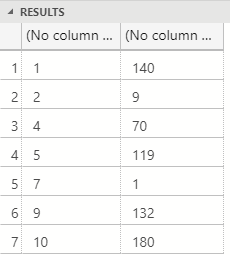Figure 5: Null Rows Not Included

We see in Figure 5 how the rows with null values are excluded from the dataset, how cool is that?! However, what about if we want null values back, like the result of this code:

``````DECLARE @x int = NULL;
DECLARE @y int = 21;

SELECT @x * @y;
``````

Code Snippet 10: Multiplication with NULL

When we run the code in Code Snippet 10 we get a result of `NULL`, and that is expected in SQL Server: any operations against a `NULL` value, yields `NULL`. To “mimic” that behaviour we use `outputNullMap`.

outputNullMap

The `outputNullMap` variable is like `inputNullMap` but the opposite. What do I mean with that: remember how the `inputNullMap` variable gets populated by the Java extension components, and read by the code we write. For the `outputNullMap,` our code populates the variable and the Java extension components read it.

To see how this works we incorporate some of the code in Code Snippet 9, with the code in Code Snippet 8, and we create a new method `multiplier3`:

``````public static void multiplier3() {

int numRows = inputDataCol1.length;
int numCols = inputNullMap.length;

outputDataCol1 = new int[numRows];
outputDataCol2 = new int[numRows];

Boolean colIsNull;

numberOfOutputCols = 2;
outputNullMap = new boolean[numberOfOutputCols][numRows];

for(int i = 0; i < numRows; i++) {
colIsNull = false;
for(int y = 0; y < numCols; y++) {
if(inputNullMap[y][i] == true) {
colIsNull = true;
break;
}
}
outputDataCol1[i] = inputDataCol1[i];
outputDataCol2[i] = inputDataCol2[i] * inputDataCol3[i];
outputNullMap[i] = false;
outputNullMap[i] = colIsNull;
}
}
``````

Code Snippet 11: Output Null Values

Some things to notice in Code Snippet 11:

• We initialise the output column arrays to the same size as the input data. We no longer need to dynamically size the output arrays, as they will contain all data passed in.
• When we loop the input rows, we also loop the `inputNullMap` and set the `colIsNull` variable to whatever the `inputNullMap` value is.
• Regardless if `colIsNull` is `true` or `false` we do the multiplication. I know, we could skip this if we have a null, but I am lazy.
• We set the value for the first column in `outputNullMap` to always be false as it represents the primary key of the data.
• The `outputNullMap` value for the second column (the result) gets the value of `colIsNull`. In other words, if a column in the row is null, we set the result column in the `outputNullMap` to be null.

After compiling etc., we execute the code in Code Snippet 5, pointing in the `@script` parameter to our new method. The result looks like so: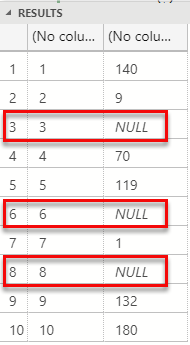Figure 6: Null Passed Back

In Figure 6 we see null values in the result set, as we would expect in an SQL Server environment. The reason we see null values (instead of 0’s) is that the Java extension components, as I mentioned before, reads the data returned together with the null map, and creates a SQL Server result set, including null values.

#### String and Null Maps

Ok, we have now seen how we use the `inputNullMap` and `outputNullMap` to handle null values, and we know we need to use the null maps for primitive types. However, what about strings, as a string is not a primitive type, and is allowed to be null in Java. Let us have a look.

NOTICE: When we push string data from SQL Server, the SQL data type has to be either `nchar` or `nvarchar`.

We create a new class, (in its own source file), in which we expect a two column data set passed in, and a two column data set returned. We also create a method which looks more or less the same as the `bar` method in Code Snippet 6:

``````public class NullStringValues {
static public int[] inputDataCol1 = new int;
static public String[] inputDataCol2 = new String;
static public boolean[][] inputNullMap = new boolean;

static public int[] outputDataCol1;
static public String[] outputDataCol2;
static public boolean[][] outputNullMap;

static public short numberOfOutputCols;

public static void bar() {

int numRows = inputDataCol1.length;
int numCols = inputNullMap.length;
for(int x = 0; x < numRows; x++) {
System.out.printf("RowID: %d\t, StringValue: %s\t,
NullMapValueStringCol: %b\n",
inputDataCol1[x],
inputDataCol2[x],
inputNullMap[x]);

}
}
}
``````

Code Snippet 12: String and Input Null Map

The code in Code Snippet 12 should not come as a surprise. We see how we:

• Have two input column arrays of which one is a `String` array.
• Also have two output column arrays of which one is a `String` array.
• In the `bar` method loops the rows, and prints out the column values as well as the value of the `inputNullMap` for the string column.

We compile the code in Code Snippet 12 and move the `.class` file to the `CLASSPATH` location. We then use SQL code like this:

``````EXEC sp_execute_external_script
@language = N'Java'
, @script = N'NullStringValues.bar'
, @input_data_1 = N'SELECT RowID, Col1
FROM dbo.tb_NullRand10
WHERE RowID IN(4, 5, 8, 9, 10)';
``````

Code Snippet 13: SQL Code for String Input

The code in Code Snippet 13 points to the `bar` method in the `NullStringValues` class, and it pushes in five rows of which two have a null value in the string column (`Col1`). The result when we execute the code in Code Snippet 13 is: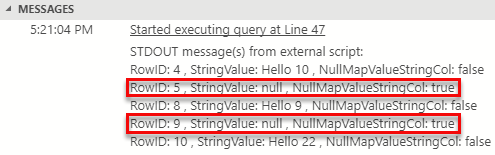Figure 7: String Nulls Passed In

We see in Figure 7 how we receive null values for the rows where the column was null. However, we also see that the null map value for those columns is set to true. So even for strings, it looks like the null map is used, at least for input data.

So, what about output data. Let us create a new method which populates the output column arrays with some random data:

`````` public static void foo() {

int numOutRows = 3;
outputDataCol1 = new int[numOutRows];
outputDataCol2 = new String[numOutRows];

numberOfOutputCols = 2;

outputNullMap = new boolean[numberOfOutputCols][numOutRows];

for(int n = 0; n < numOutRows; n++) {
outputDataCol1[n] = n+1;
outputNullMap[n] = false;
if(n == 1) {
outputDataCol2[n] = null;
outputNullMap[n] = true;
}
else {
outputDataCol2[n] = "Hello";
outputNullMap[n] = false;
}

}
}
``````

Code Snippet 14: Java Code for String Null Output

We see in Code Snippet 14 how we output three rows with two columns, where the second column is a string. In the second row, the string column is set to `null`, and the `outputNullMap` is also set to `null` for that column. After compilation, etc. we use following SQL code:

``````EXEC sp_execute_external_script
@language = N'Java'
, @script = N'NullStringValues.foo';
``````

Code Snippet 15: SQL Code for String Output

The code runs fine when we execute, and the result looks like so:Figure 8: String Nulls Passed Out

As expected we get a null value back in the outlined row in Figure 8. This does not tell us though whether the `outputNullMap` is required for strings. Let us comment out all code inside the `for` loop that references the `outputNullMap`. Keep the initialization of the null map as is (`outputNullMap = new boolean[numberOfOutputCols][numOutRows];`), compile, etc. Remember that for output data sets with no nulls we only need to initialise the `outputNullMap`. The theory is that for non-primitive data types we do not need to set the null map.

When we now execute the code in Code Snippet 15 we get an error:Figure 9: String Nulls without Output Null Map

Ok, so the theory above does not seem correct based on the exception we see in Figure 9. So what about if the column is null, but the `outputNullMap` says `false` (not null):

``````if(n == 1) {
outputDataCol2[n] = null;
outputNullMap[n] = false;
}
``````

Code Snippet 16: String Value Null Null Map False

When we execute after having compiled the code after the change in Code Snippet 16, we get the same exception as we see in Figure 9. Also, if we assign a value to the string column, but set the `outputNullMap` to be `true`:

``````if(n == 1) {
outputDataCol2[n] = "xxx";
outputNullMap[n] = true;
}
``````

Code Snippet 17: String Value Null Null Map False

When we execute the code in Code Snippet 17 we get:Figure 10: String not Null with Output Null Map True

Hmm, that is interesting! We see in Figure 10 how we do receive data back, but also an exception, and notice that the exception has a different `HRESULT` than the exception in Figure 9.

NOTICE: I do believe that Microsoft can do a better job with the errors returned from the Java extensions, as they at the moment are not very descriptive.

We do not receive all rows, the row where the actual string value is not null but the null map says it is, is the last row. Oh, and the value for the column is what the null map say - `null`.

We now know that the null maps are needed for strings as well as primitive types. It also seems like the Java extensions are doing some validation when data returns from Java.

## Summary

Wow, this turned out to be quite a long blog post! So what have we found out:

When we deal with data passing in Java code we need two null maps:

• `static public boolean[][] inputNullMap`: for input data.
• `static public boolean[][] outputNullMap`: for output data.

You initialize the `inputNullMap`, and the Java extensions components populate the map, based on the data pushed in from SQL Server. You initialise and populate the `outputNullMap`, and the Java extensions components read the map and create the resultset returned to SQL Server. Even for string null values you need to populate the `outputNullMap`.

In your code, you can read the `inputNullMap` to decide how to process the data pushed in.

## ~ Finally

If you have comments, questions etc., please comment on this post or ping me.